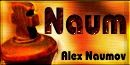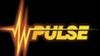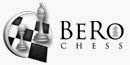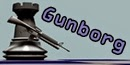## Posts

Showing posts from September, 2014

### Since you’re here...

... we have a small favour to ask. More people, like you, are reading and supporting our blog: "Chess Engines Diary". And unlike many other sites and blogs, we made the choice to keep our articles open for all, regardless of where they live or what they can afford to pay.

We hope you will consider supporting us today. We need your support to continue to exist, because good entries are more and more work time. Every reader contribution, however big or small, is so valuable. Support "Chess Engines Diary" even a small amount– and it only takes a minute. Thank you.

================================================
My email: jotes@go2.pl### Equinox 3.2 free!Equinox - UCI chess engine Author - Giancarlo Delli Colli Equinox 3.2 free - download

### Naum 4.6 - free!Naum - UCI chess engine Author - Alex Naumow Naum 4.6 (free) - download

### DeepJunior Yokohama wins 2 League Jurek Chess Engines Rating - Season 8/2014

JCER 2 League, season 8/2014, 2014.09.26 - 2014.09.30 Player JCER Score De De Sp Fr To EX Ga Ry Mi Ar Bo To De Ne Di Cr Chg 1: Deep Junior Yokohama x64 2786 21.0 / 30 X =1 01 =1 0= 1= =0 01 1= 11 11 0= 11 1= 11 11 +45 2: DeepHiarcs14WCSC 2906 19.5 / 30 =0 X 01 1= =1 11 11 10 =0 00 == =1 1= 11 11 1= -21 3: Spark 1.0 x64 2801 17.5 / 30 10 10 X 01 =0 0= 0= =1 =0 11 10 =1 10 11 =1 11 +3 4: Fruit reloaded 2717 17.5 / 30 =0 0= 10 X 0= 10 11 0= == =1 =1 01 1= =1 11 =1 +42 5: Toga II 280513 x64 2736 17.0 / 30 1= =0 =1 1= X =0 00 01 11 11 10 =1 10 =1 10 =0 +30 6: EXchess 7.31b x64 2708 17.0 / 30 0= 00 1= 01 =1 X 1= 1= 11 10 =0 11 == 10 10 == +45 7: Gaviota 1.0 x64 2689 16.5 / 30 =1 00 1= 00 11 0= X == == 00 =1 10 =1 01 11 11 +45 8: Rybka 2.3.2a 64

### Amaterasu r22 - winboard chess engineAmaterasu - winboard chess engine Author - Bogdan Mihail Claudin All engines downloadable on the site

### Animats r.23 winboard chess engineAnimats - winboard chess engine Author - Stuart Allen All engines downloadable on the site

### Alf 1.09 - winboard chess engineAlf - winboard chess engine Author - Casper W. Berg   All download from the site

### Alarm 0.93.1, winboard chess engine - new logoAlarm - winboard chess engine Authors: Benny Antonsson and Erik Robertsson (Sweden) Alarm 0.93.1 download

### Celes, UCI chess engines - new logoCeles - UCI chess engines Author - Johan Hutting Celes 0.77c - downloadable on the site

### Pulse 1.5.0 - winboard chess engine. New version 28.09.2014Pulse - winboard chess engine Author -  Phokham Nonava  Pulse 1.5.0 download

### Berochess, UCI chess engine - new logoBerochess - UCI chess engine Author - Benjamin Rosseaux     Berochess downloadable on the site

### Eden, UCI chess engine - new logoEden - UCI chess engine Author - Nicolai Czempin Eden 0.0.13 download

### Gunborg, UCI chess engine - new logo### Stockfish 14092722 - new development version strong chess engine UCIStockfish  - chess engines UCI Author compilation - Uri Blass   Stockfish 14092722 download

### TCEC Season 7 - live 27.09.2014

N Engine Rtng Pts Gm SB Gu Ci Ha St Ch Cr Pr Sp Ga Tg To Ar Oc Al 1 Gull 3 3033 5.0 6 15.00 ·· 1 1 = 1 1 = 2 Chiron 2 2955 4.5 6 10.25 0 ·· 1 1 1 = 1 3 Hannibal 1.5x5 2924 4.5 6 8.50 ·· = = 1 1 = 1 4 Stockfish 160914 3168 4.0 6 7.25 0 ·· = = 1 1 1 5 Cheng4 0.36c+ 2779 4.0 6 7.25 = ·· 0 = 1 1 1 6 Critter 1.6a 3030 3.5 6 9.50 = 0 = ·· = 1 1 7 Protector 1.7 2914 3.0 6 9.75 0 = 1 = ·· = = 8 Spike 1.4 2876 3.0 5 8.00 = = ·· = 1 = 9 Gaviota 1 2691 2.0 6 4.00 0 0 0 0 ·· 1 1 10 Toga II 010214 2699 2.0 6 4.00 0 = = 0 ·· = = 11 Tornado 5 2778 2.0 6 3.00 0 = 0 0 ·· 1 = 12 Arasan 17.3 2525 1.5 6 6.25 =

### Elektro 1.0a (Igorrit derivate) UCI chess engine. New version 27.09.2014Elektro  - UCI chess engines Author - Otto Schmidt.       Elektro 1.0a download

### Critter wins 1 League Jurek Chess Engines Rating - Season 8/2014

JCER 1 League, season 8/2014, 2014.09.24 - 2014.09.26 P Engines JCER Score Cr Eq De Pa Iv Pr Bl Fi Ha Se De Ch Ar Sp Te Za Chg 1: Critter 1.6a x64 3046 23.0 / 30 X == =1 == 1= == == 11 =1 =1 11 11 11 1= 11 1= +12 2: Equinox 2.02 x64 2983 22.0 / 30 == X =0 == 11 1= 1= == =1 1= 1= 11 11 11 1= 1= +27 3: Deep Rybka 4.1 x64 2985 21.0 / 30 =0 =1 X =0 =1 =1 =0 1= 1= 11 == =1 =1 11 11 11 +18 4: PanChess 00.611 x64 3012 20.0 / 30 == == =1 X 0= =0 =1 == =1 1= == 1= 1= 11 11 11 -3 5: IvanHoe 46h x64 3013 18.0 / 30 0= 00 =0 1= X == == =1 10 =1 =1 == =1 1= 11 11 -24 6: Protector 1.7 x64 2871 16.5 / 30 == 0= =0 =1 == X == == 10 =0 1= 01 01 1= 1= 11 +24 7: BlackMamba 2.0 x64 2929 16.5 / 30 == 0= =1 =0 == == X == =0 00 01 11 1= 11 =1 1= -3 8: Firenzina 2.4.2 xTreme 2985

### Sugar - new chess engine (clone Stockfish)Sugar - UCI chess engine (clone Stockfish) Author - Marco Zerbinati Sugar download

### Stockfish 14092521 - new development version strong chess engine UCIStockfish - chess engines UCI  Author compilation - Joona Kiiski    Stockfish 14092521 download

### Elektro 1.0 (Igorrit derivate) - new chess engineElektro - UCI chess engines Author - Otto Schmidt.       Elektro 1.0 download

### Stockfish wins Super League JCER - Season 8/2014

JCER Super League season 8/2014, 2014.09.22 - 2014.09.24 P Engines JCER Score St He Ho Bo Gu Am Ko St DO Ko Ho Co Ma Fi De Ro Chg 1: Stockfish 14091916 x64 3227 21.0 / 30 X == 1= == =1 == =1 == == =1 == 1= =1 11 11 11 -12 2: Heron impossible 280814 3100 20.0 / 30 == X =1 1= == == =0 == 11 =1 1= 1= 11 =1 11 0= +30 3: Houdini 4 x64A 3143 18.5 / 30 0= =0 X 1= == == 11 =1 == =1 01 == =1 =1 1= =1 -3 4: Bouquet 1.7 x64 2998 17.5 / 30 == 0= 0= X =1 == 0= 01 1= 10 =1 11 =1 01 == 11 +51 5: Gull 3 x64 3090 17.5 / 30 =0 == == =0 X =0 1= =0 == 1= 01 =1 1= 11 11 =1 +6 6: Amitis 2013-09-12 3000 16.5 / 30 == == == == =1 X 00 =1 1= == =1 == =1 =0 1= == +42 7: Komodo 8 x64 3158 16.5 / 30 =0 =1 00 1= 0= 11 X =0 == =1 == =1 == =1 =1 == -30

### Fizbo 1.2 winboard chess engineFizbo  - winboard chess engine Author -  Youri Matiounine Fizbo 1.2 download

### Mars 3.3 - (strong) chess engines UCI (based on Ippolit) . New version 22.09.2014Mars  - UCI chess engines    Older version    JCER  : Mars 2,1 = 3062, 3014 Mars Mars 2,8 = 1,5 = 3035, Mars 1,7 = 3018, Mars 2,0 = 3015, Mars 1,1 = 2981 ,  Mars 1,8 = 3024, Mars 3,1 = 3022,  Mars 1,6 = 2886,   Mars 3.3 download

### Rhetoric 1.4.1 - UCI chess engine. New version 22.09.2014Rhetoric  - UCI chess engines Author - Alberto Sanjuan Rating JCER older versions - Rhetoric 1.2=2556, Rhetoric 1.1=2445, Rhetoric 1.0=2404 Rhetoric 1.4.1 download

### Sjakk 2.2 UCI chess engine. New version 22.09.2014 - and first test JCERSjakk  - UCI chess engine Author -  Jacob Børs Lind JCER ratings older version: Sjakk 2.0 = 2305, Sjaak 2.1 download JCER - First Test Sjakk 2.2, CHESSENGINES, 2014.09.21 - 2014.09.22 Engines JCER Score Sjakk 2.2 Chg +/-/= 1: Sjakk 2.2 x64 2400 62.0 / 100 XXXXXXXX +150 (+51 -27 =22) 2: Arasan 17.3 x64 2740 9.0 / 10 1111111=1= +1 (+8 -0 =2) 3: Gaviota 1.0 x64 2689 8.5 / 10 11=11=11=1 +0 (+7 -0 =3) 4: Pedone 0.5 x64 2277 8.0 / 10 1=1111110= +47 (+7 -1 =2) 5: Gojemat 6.6.1.b01 2559 4.5 / 10 1=01=001=0 -27 (+3 -4 =3) 6: Cheese 1.61 x64 2499 3.5 / 10 =0=0==0=== -29 (+0 -3 =7) 7: Gibbon 2.60a x64 2352 2.0 / 10 0000000101 -23 (+2 -8 =0) 8: NGplay 9.86 x64 2469 1.0 / 10 =000000=00 -50 (+0 -8 =2) 9: K2 0.63 2355 1.0 / 10 000000==00 -33 (+0 -8 =2) 1

### Jurek Chess Engines Rating: launched Super League (season 8/2014)JCER 3 League (season 7/2014) Temp 3' + 3", GUI Arena 3.5 Engines:  Heron impossible 280814,  Stockfish 14091916, Mars 2.1,  Bouquet 1.7,  Amitis 2013-09-12,  Strelka 6,  Komodo 8,  Komodo 5.1r2,  Houdini 1.5a,  Houdini 4 x64,  DeepSaros eXp 6h,  Gull 3,  Fire 3.0,  ComStock 3,  RobboLito 0.21Q,  DON 050914a

### Jurek Chess Engines Rating: first test Protector 1.7

JCER First Test Protector 1.7, CHESSENGINES, 2014.09.20 - 2014.09.21 Player JCER Score Protector Chg +/-/= 1: Protector 1.7 x64 2860 50.0 / 100 XXXXXXXX -30 (+33 -33 =34) 2: BlackMamba 2.0 x64 2927 8.0 / 10 1101111011 +13 (+8 -2 =0) 3: PanChess 00.611 x64 3024 8.0 / 10 =1011111=1 +4 (+7 -1 =2) 4: Houdini 1.5a x64 3035 7.5 / 10 ====11=111 -2 (+5 -0 =5) 5: Critter 1.4 x64 3050 6.5 / 10 011=01==11 -14 (+5 -2 =3) 6: RobboLito 0.21Q x64 3005 6.5 / 10 11==1==01= -10 (+4 -1 =5) 7: Protector 1.6.0 x64 2860 5.5 / 10 =1=1=====0 -5 (+2 -1 =7) 8: Arasan 17.3 x64 2748 2.5 / 10 =000101000 -22 (+2 -7 =1) 9: Protector 1.7.0 beta r938 x64 2860 2.5 / 10 000=0====0 -35 (+0 -5 =5) 10: EXchess 7.3

### Jurek Chess Engines Rating - new ranking list 21.09.2014All list

### Stockfish 14091916 - new development version strong chess engine UCI and first test Jurek Chess Engines RatingStockfish - chess engines UCI  Author compilation - " mbootsector "    Stockfish 14091916 download JCER Test Stockfish 14091916, 2014.09.19 - 2014.09.20 P Engines JCER Score Stockfish Chg +/-/= 1: Stockfish 14091916 x64 3196 58.0 / 100 XXXXXXXX -110 (+22 -6 =72) 2: Stockfish 14072002 x64 3193 5.0 / 10 ========== -10 (+0 -0 =10) 3: Stockfish 14083022 x64 3196 5.0 / 10 01=10==01= -10 (+3 -3 =4) 4: Stockfish 14091622 x64 3196 5.0 / 10 ========== -10 (+0 -0 =10) 5: Stockfish 5 x64 3143 5.0 / 10 ========== -4 (+0 -0 =10) 6: Critter 1.6a x64 3030 4.0 / 10 ===01=00== -1 (+1 -3 =6) 7: Komodo 8 x64 3131 4.0 / 10 ==0=0=0=1= -13 (+1 -3 =6) 8: Deep Rybka 4.1 x64 2985 3.5 / 10 0=0===0=== -1 (+0 -3 =7) 9: Stockfish 14071112 x64 3179 3.5 / 10 ==0=0=0===

### League Season 7/2014 - Jurek Chess Engines Rating - completedLeague Season 7/2014 - Jurek Chess Engines Rating - completed

### Amitis wins 4 League JCER - Season 7/2014

JCER 4 League 7/2014, 2014.09.18 - 2014.09.20 Player JCER Score Am Jo An At Re Go Gr Dj Ch Tu Pr Ma Gi NG Sa Da Chg 1: Amitis 2013-09-12 2594 29.5 / 30 X 11 11 =1 11 11 11 11 11 11 11 11 11 11 11 11 +99 2: Jonny 4.0 2472 23.0 / 30 00 X 0= == =1 11 11 0= 11 11 1= 11 11 11 11 11 +93 3: Andscacs 0.64 2506 20.5 / 30 00 1= X =1 11 =1 =1 01 01 11 1= 00 10 11 11 11 +48 4: Atlas 3.70em x64 2563 20.0 / 30 =0 == =0 X =0 11 00 =1 1= 1= 01 11 11 11 11 11 +18 5: Redqueen 1.1.97 x64 2500 19.5 / 30 00 =0 00 =1 X 11 10 1= =1 11 =1 00 11 11 11 11 +42 6: Godel 3.4.9 x64 2542 18.0 / 30 00 00 =0 00 00 X 11 11 =1 =1 1= 11 01 11 11 11 +6 7: GreKo 12.0 x64 2480 16.0 / 30 00 00 =0 11 01 00 X =0 =0 11 10 11 1= 10 11 11 +18 8: Djinn1.021 x64 2589 16.0 / 30 00 1= 10 =0 0= 0